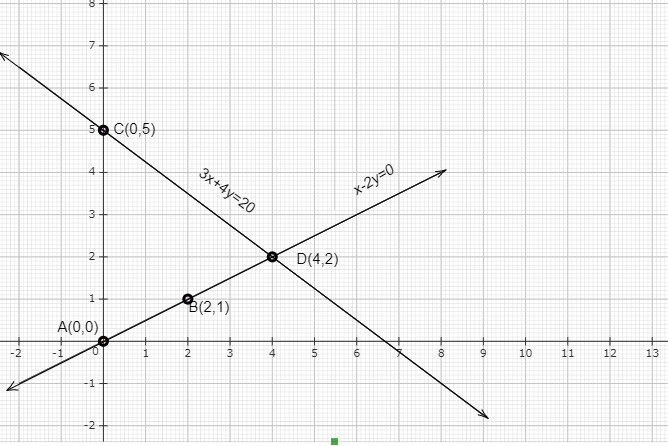# Akhila went to a fair in her village. She wanted to enjoy rides on the Giant Wheel and play Hoopla (a game in which you throw a rig on the items in the stall, and if the ring covers any object completely you get it). The number of times she played Hoopla is half the number of rides she had on the giant wheel. Each ride costs √Ę‚Äö¬Ļ3 and a game of hoopla costs √Ę‚Äö¬Ļ4. If she spent √Ę‚Äö¬Ļ20 in the fair, represent this situation algebraically and graphically.

Given:

The number of times Akhila played Hoopla is half the number of rides she had on the giant wheel.

Cost of each ride$=Rs.\ 3$

Cost of a game of hoopla$=Rs.\ 4$.

Total money spent in the fair$=Rs.\ 20$.

To do:

We have to represent the above situation algebraically and graphically.

Solution:

Let $x$ be the number of rides Akhila had on the giant wheel and $y$ be the number of times she played Hoopla.

According to the question,

$y = \frac{1}{2}x$

$\Rightarrow x-2y = 0$.....(i)

$3x + 4y = 20$......(ii)

$4y=20-3x$

$y=\frac{20-3x}{4}$

To represent the above equations graphically we need at least two solutions for each of the equations.

For equation (i),

If $x=0$ then $y=\frac{0}{2}=0$

If $x=2$ then $y=\frac{2}{2}=1$

 $x$ 0 2 $y=\frac{1}{2}x$ 0 1

For equation (ii),

If $x=0$ then $y=\frac{20-3(0)}{4}=5$

If $y=0$ then $0=\frac{20-3x}{4}$

$\Rightarrow 20=3x$

$\Rightarrow x=\frac{20}{3}$ which is not an integer so it is not easy to plot on graph paper.

So, let us take $y=2$

If $y=2$ then $2=\frac{20-3x}{4}$

$\Rightarrow 20-3x=8$

$\Rightarrow 3x=20-8$

$\Rightarrow x=\frac{12}{3}$

$\Rightarrow x=4$

 $x$ 0 $\frac{20}{3}$ 4 $y=\frac{20-3x}{4}$ 5 0 2

The above situation can be plotted graphically as below:The line AB represents the equation $x-2y=0$ and the line CD represents the equation $3x+4y=20$.

The two lines intersect at D(4,2) which is a solution of both the equations.

Updated on: 10-Oct-2022

103 Views